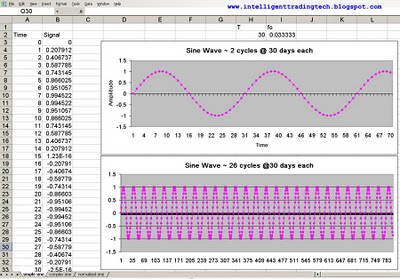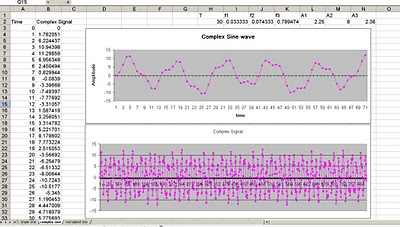Want to share your content on R-bloggers? click here if you have a blog, or here if you don't.

The following introduction is to allow viewers to understand the basic concepts and practical implementation of neural nets towards a financial time series. I will not go too deep into detail about the mathematics behind the neural net at the moment. My goal is to get you to understand practical details about how to actually implement a neural net using simple tools and models. We will start with a simple model to understand a basic time series. The time series waveform is a simple sine wave with the period set to 30 days. It is implemented in excel as a source file to be processed in any Machine Learning capable software. For this example I will be using a very good GUI Java based program called Weka.Fig 1. Shows a simple sine wave set to a period (T) of 30 days.

It is a very simple time series based upon the well known sine wave model.
We can see that one complete cycle occurs over a period of 30 days. Each time step is set to 1 unit or day per step.Fig 2. A complex sinusoidal signal with f1 set to 1/T, where T=30 days.

Anyone who has worked with financial time series knows that they can be far more complicated than simple sine based models, however, it is often better to learn from basic principles and move up in complexity in order to have a good grasp of what we are doing. The second figure is a bit more complicated as it is the sum of three different sin based signals. Each signal has a different Amplitude and Frequency associated with it. We could use Fourier Analysis to show the spectrum of the three different tones if we wished. However, for now we’ll just accept that it is a complex signal. Notice one property of this signal that is also a bit optimistic is that it is a stationary signal. Essentially a stationary signal has statistical properties that do not change over time. For example, if we were to sample the average from different slices, it would not change much. We also can visually see that the time series is mean reverting. Financial time series differ in that they are not stationary, but are typically unit root and must often be transformed in order for the neural network to process them. The purpose of the complex signal, however, is to show how we can move to an increasingly complex signal from a very simple model.Fig 3. Normalized Complex Signal

The final step is to simply normalize the time series to be constrained between the vertical (what we call rails) range of minus 1 to plus 1. A typical neural net is limited by an internal function, sometimes called a squashing function. The function is a non-linear processing function that is often made up of a sigmoid or tanh (hyperbolic tangent) function, which saturate at (0,1) and (-1,1), respectively.
A simple transformation can be produced by xnew =xold*(vmaxnvminn)/(vmaxovmino).
Vmax and Vmin are the new and old maximum values of the time series. In this case we will use -.9 and +.9 as the limiting rails so as to avoid saturation effects. Often software will do the normalizing for you. In the case of Weka, you can choose to have it do this operation for you, in which case no normalization is neccessary. Although we should understand it for future reference.

That’s it for part I. Next we will investigate how to transport the data to Weka and have it build and predict the out of sample signal set!

Please add any comments on where I can improve my tutorial as I am new to the blogger scene and appreciate any feedback.